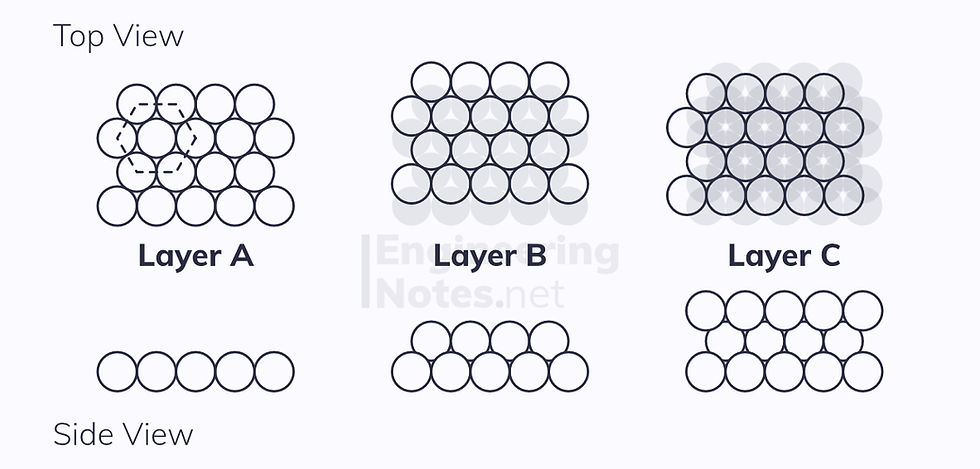top of page

#### Notes by Category University Engineering

Rate these notesNot a fanNot so goodGoodVery goodBrillRate these notes

# Crystal Structures

In this notes sheet:

Understanding the molecular structure of a material is crucial in understanding how that material behaves. Many of its physical properties are directly caused and explained by its crystalline structure (known as a lattice). We define this as:

A crystalline lattice is a regular repeating pattern of atoms or molecules.

An amorphous material is one that does not display a crystalline structure. Instead, the structure is random – examples include some polymers and ceramics.

• Some other polymers are known as semi-crystalline

## The Hard Sphere Model

The Hard Sphere Model allows us to model atoms or molecules as, you guessed it, hard spheres. There are a number of conditions for this to apply:

1. The outer electron shell must be full

2. The remaining electrons must be distributed rotationally symmetrically

3. Electron clouds of neighbouring atoms do not overlap much or at all

For the outer shell of electrons to be full, metals must lose electrons, while non-metals must gain electrons. This means they are actually ions.

## Packing in Two Dimensions

It is impossible to arrange circles in such a way that there are no unfilled spaces between them:Squares, rectangles, triangles, and hexagons, however, can be arranged into a complete lattice:• The primitive unit cell is the smallest cell that can be regularly repeated throughout the structure – the hexagon can be split into six equilateral triangles.

• The unit cell is the easiest cell to repeat: the hexagonal is more practical, as it does not require rotating, whereas the triangle does.

The two arrangements of circles above can, however, be modelled as square and rectangular patterns respectively, where the centre of an atom is at each vertex:Shown in red on the diagrams are the close-packed directions of each structure. These are the lines along which ions continuously touch (the line never passes through empty space). As you can see:

• The square lattice has two close-packed dimensions

• The hexagonal lattice has three close-packed directions

Since the hexagonal lattice is more densely packed, it is known as the close-packed arrangement.

## Packing in Three Dimensions

If we look at the same arrangements in 3D, is clear that, as well as close-packed directions, there are close-packed planes. These are planes in which all the ions are arranged in a hexagonal lattice.

There are four typical unit cells in three dimensions:

### Simple Cubic

This is simply a 2D square lattice in 3D:• 1 atom per unit cell (⅛ on each vertex)

• 6 nearest neighbours (each ion touches six others - also known as the coordination number)

• Lattice parameter: a = 2r

• No close-packed planes

• No slip systems

• Atomic packing factor: 52.4%

### Body-Centred Cubic (BCC)

In the simple cubic cell above, there is a gap in the centre. When the outer atom eights are moved apart enough to fit another atom in the middle, you have the BCC arrangement:• 2 atoms per unit cell (⅛ at each vertex, one in the centre)

• 8 nearest neighbours

• Lattice parameter a = ⁴/₃ √3 r

• No close-packed planes

• 2 close-packed directions

• No slip systems

• Atomic packing factor: 68.2%

### Face-Centred Cubic (FCC)

This is the most densely packed cubic arrangement. In this instance, there are three close-packed planes stacked on top of one another, in an ABC arrangement:Again, the unit cell is a cube, but the atoms on the vertices are further apart. This allows 4 half atoms to fit in the centre:• 4 atoms per unit cell (⅛ on each vertex, ½ on each side)

• 12 nearest neighbours